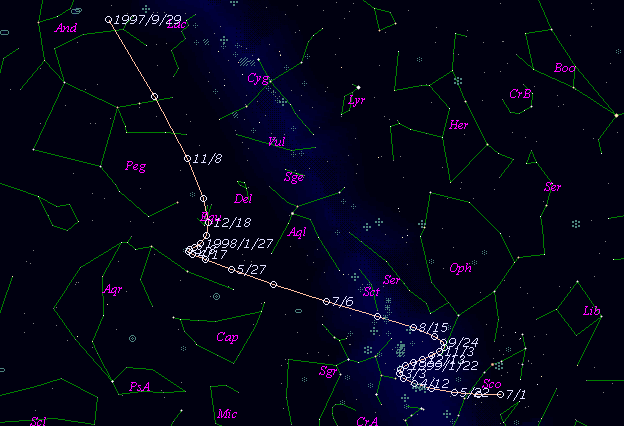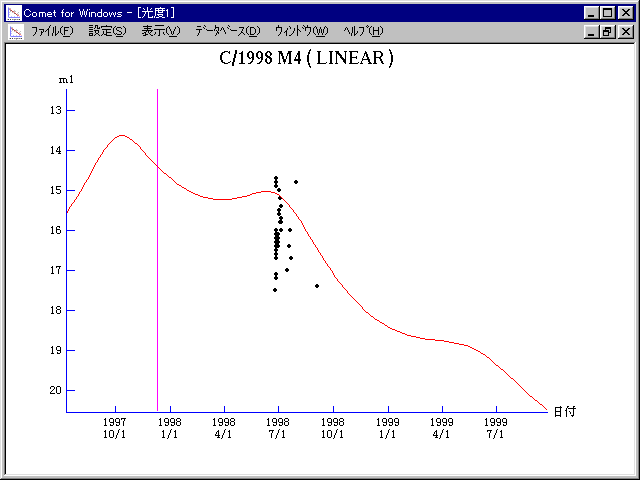# \$B%j%K%"WB@1(B

C/1998 M4 ( LINEAR )###\$B%W%m%U%#!<%k(B

 \$BH/8+F|(B 1998\$BG/(B6\$B7n(B25\$BF|(B \$BH/8+8wEY(B 17.5\$BEy(B \$BH/8+ Lincoln Laboratory Near-Earth Asteroid Research project

###\$B50F;MWAG(B

```   The following improved orbital elements by Kenji Muraoka are
from 115 observations  1998 June 25 to Sept. 4, perturbations
by 9 Planets, Moon and 5 minor planets were taken into account.
The mean residual is +/- 0.70 arc seconds.

Epoch  =  1997 Dec. 18.0  TT       JDT = 2450800.5
T  =  1997 Dec. 10.29421       +/- 0.08230 (m.e.) TT
Peri. =  106.31401                +/- 0.02029
Node  =   92.90047                +/- 0.02521   (2000.0)
Incl. =  154.58216                +/- 0.01235
q  =    2.6007208              +/- 0.0004488 AU
e  =    0.9986194              +/- 0.0007039
1/a  =   +0.0005309              +/- 0.0002707 1/AU
( P  =  81758 years )
```

###\$B@1?^(B###\$B8wEYJQ2=(B

```        m1 = 8.0 + 5 log\$B&\$(B + 10.0 log r
```##### \$B50F;MWAG\$OB<2,7r<#;a\$N7W;;\$K\$h\$k\$b\$N\$G\$9!#(B \$B@1?^\$O(B StellaNavigator Ver.2.0 for Windows (\$B%"%9%H%m%"!<%D(B \$BJTCx(B / \$B%"%9%-!<=PHG6I4)(B) \$B\$G:n@.\$7\$?\$b\$N\$G\$9!#(B \$B8wEY%0%i%U\$O(BComet for Windows\$B\$G:n@.\$7\$?\$b\$N\$G\$9!#(B